# CBSEtips.in

## Thursday, 28 January 2021

### CBSE Class 10 Maths - MCQ Questions and Online Tests - Unit 10 - Circles

#### CBSE Class 10 Maths – MCQ and Online Tests – Unit 10 – Circles

Every year CBSE conducts board exams for 10th standard. These exams are very competitive to all the students. So our website provides online tests for all the 10th subjects. These tests are also very effective and useful for those who preparing for competitive exams like NEET, JEE, CA etc. It can boost their preparation level and confidence level by attempting these chapter wise online tests.

These online tests are based on latest CBSE Class 10 syllabus. While attempting these our students can identify the weak lessons and continuously practice those lessons for attaining high marks. It also helps to revise the NCERT textbooks thoroughly.

#### CBSE Class 10 Maths – MCQ and Online Tests – Unit 10 – Circles

Question 1.
If radii of two concentric circles are 4 cm and 5 cm, then the length of each chord of one circle which is tangent to the other circle is
(a) 3 cm
(b) 6 cm
(c) 9 cm
(d) 1 cm

Question 2.
In Fig., if ∠AOB = 125°, then ∠COD is equal to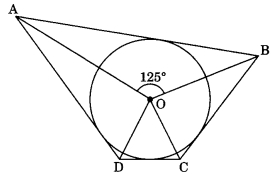(a) 62.5°
(b) 45°
(c) 35°
(d) 55°

Question 3.
If Fig., AB is a chord of the circle and AOC is its diameter such that ∠ACB = 50°. If AT is the tangent to the circle at the point A, the ∠BAT is equal to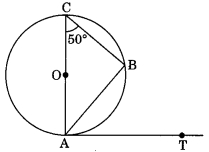(a) 65°
(b) 60°
(c) 50°
(d) 40°

Question 4.
From a point P which is at a distance of 13 cm from the point O of a circle of radius 5 cm, the pair of tangents PQ and PR to the circle are drawn. Then the area of the quadrilateral PQOR is
(a) 60 cm²
(b) 65 cm²
(c) 30 cm²
(d) 32.5 cm²

Question 5.
At one end A of a diameter AB of a circle of radius 5 cm, tangent XAY is drawn to the circle. The length of the chord CD parallel to XY and at a distance 8 cm from A is
(a) 4 cm
(b) 5 cm
(c) 6 cm
(d) 8 cm

Question 6.
In Fig., AT is a tangent to the circle with centre O such that OT = 4 cm and ∠OTA = 30°. Then AT is equal to(a) 4 cm
(b) 2 cm
(c) 2√3 cm
(d) 4√3 cm

Question 7.
In Fig., if O is the centre of a circle PQ is a chord and the tangent PR at P makes an angle of 50° with PQ, then ∠POQ is equal to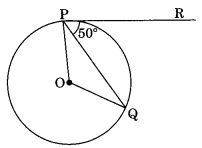(a) 100°
(b) 80°
(c) 90°
(d) 75°

Question 8.
In Fig., if PA and PB are tangents to the circle with centre 0 such that ∠APB = 50°, then ∠AOB is equal to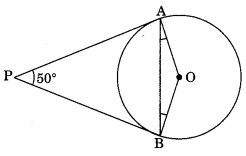(a) 25°
(b) 130°
(c) 100°
(d) 50°

Question 9.
If two tangents inclined at an angle 60° are drawn to a circle of radius 3 cm the length of each tangent is equal to
(a) $$\frac{3}{2}$$√3 cm
(b) 6 cm
(c) 3 cm
(d) 3√3 cm

Question 10.
In Fig., if PQR is the tangent to a circle at Q whose centre is O, AB is a chord parallel to PR and ∠BQR = 70°, then ∠AQB is equal to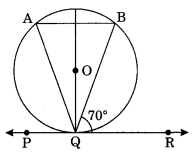(a) 20°
(b) 40°
(c) 35°
(d) 45°

Share: